# Problem Solving Strategies.pptx

Mar. 23, 2023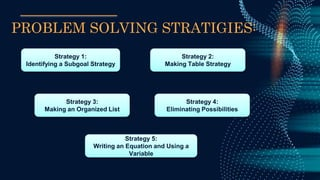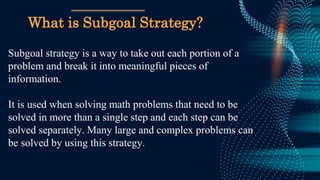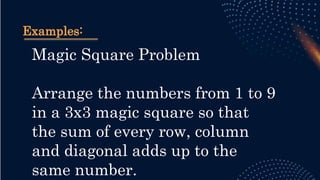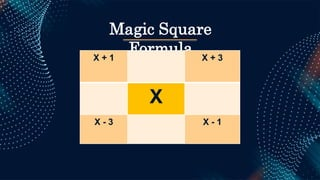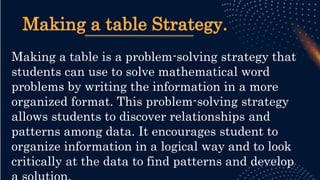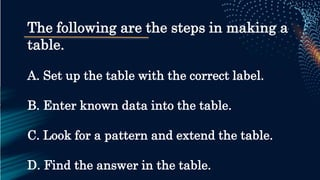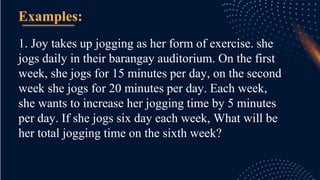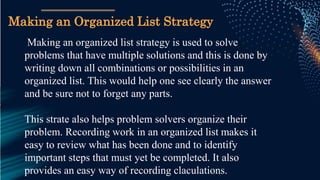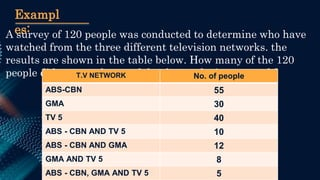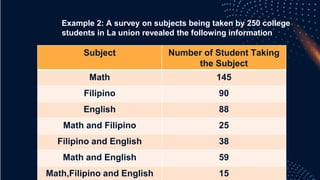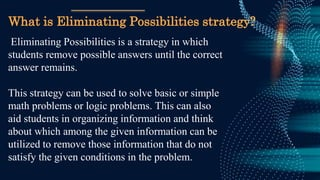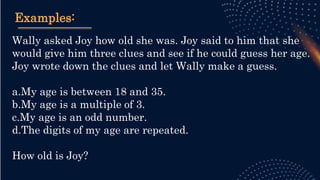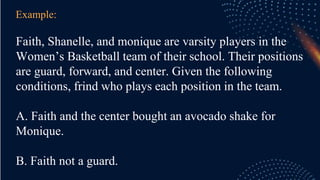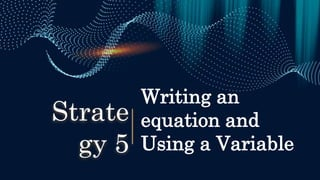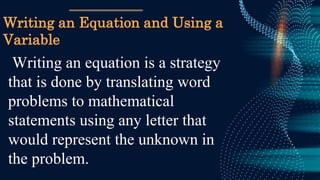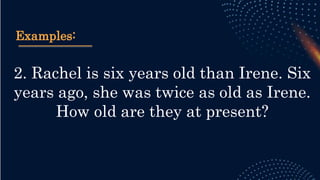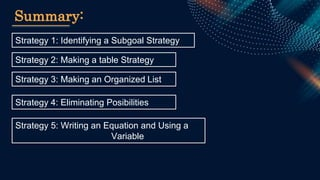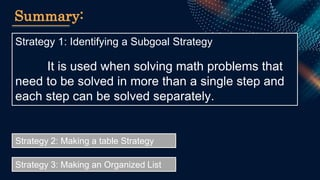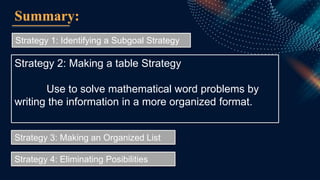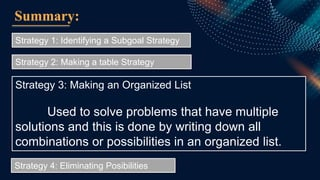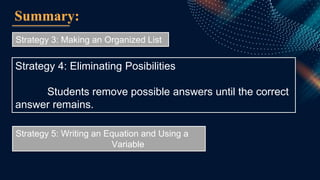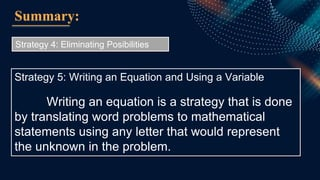1 of 31

### Problem Solving Strategies.pptx

1. Problem Solving Strategies Group 3
2. PROBLEM SOLVING STRATIGIES:
3. PROBLEM SOLVING STRATIGIES: Strategy 1: Identifying a Subgoal Strategy Strategy 2: Making Table Strategy Strategy 4: Eliminating Possibilities Strategy 5: Writing an Equation and Using a Variable Strategy 3: Making an Organized List
4. Identifying a subgoal Strategy Strate gy 1
5. What is Subgoal Strategy? Subgoal strategy is a way to take out each portion of a problem and break it into meaningful pieces of information. It is used when solving math problems that need to be solved in more than a single step and each step can be solved separately. Many large and complex problems can be solved by using this strategy.
6. Examples: Magic Square Problem Arrange the numbers from 1 to 9 in a 3x3 magic square so that the sum of every row, column and diagonal adds up to the same number.
7. Magic Square Formula X + 1 X + 3 X X - 3 X - 1
8. Making a table Strategy Strate gy 2
9. Making a table Strategy. Making a table is a problem-solving strategy that students can use to solve mathematical word problems by writing the information in a more organized format. This problem-solving strategy allows students to discover relationships and patterns among data. It encourages student to organize information in a logical way and to look critically at the data to find patterns and develop
10. The following are the steps in making a table. A. Set up the table with the correct label. B. Enter known data into the table. C. Look for a pattern and extend the table. D. Find the answer in the table.
11. 1. Joy takes up jogging as her form of exercise. she jogs daily in their barangay auditorium. On the first week, she jogs for 15 minutes per day, on the second week she jogs for 20 minutes per day. Each week, she wants to increase her jogging time by 5 minutes per day. If she jogs six day each week, What will be her total jogging time on the sixth week? Examples:
12. Making an Organized List Strategy Strate gy 3
13. Making an organized list strategy is used to solve problems that have multiple solutions and this is done by writing down all combinations or possibilities in an organized list. This would help one see clearly the answer and be sure not to forget any parts. This strate also helps problem solvers organize their problem. Recording work in an organized list makes it easy to review what has been done and to identify important steps that must yet be completed. It also provides an easy way of recording claculations. Making an Organized List Strategy
14. Exampl es: A survey of 120 people was conducted to determine who have watched from the three different television networks. the results are shown in the table below. How many of the 120 people did not watch any of the three televion network? T.V NETWORK No. of people ABS-CBN 55 GMA 30 TV 5 40 ABS - CBN AND TV 5 10 ABS - CBN AND GMA 12 GMA AND TV 5 8 ABS - CBN, GMA AND TV 5 5
15. Subject Number of Student Taking the Subject Math 145 Filipino 90 English 88 Math and Filipino 25 Filipino and English 38 Math and English 59 Math,Filipino and English 15 Example 2: A survey on subjects being taken by 250 college students in La union revealed the following information
16. Eliminating Possibilities Strate gy 4
17. What is Eliminating Possibilities strategy? Eliminating Possibilities is a strategy in which students remove possible answers until the correct answer remains. This strategy can be used to solve basic or simple math problems or logic problems. This can also aid students in organizing information and think about which among the given information can be utilized to remove those information that do not satisfy the given conditions in the problem.
18. Examples: Wally asked Joy how old she was. Joy said to him that she would give him three clues and see if he could guess her age. Joy wrote down the clues and let Wally make a guess. a.My age is between 18 and 35. b.My age is a multiple of 3. c.My age is an odd number. d.The digits of my age are repeated. How old is Joy?
19. Example: Faith, Shanelle, and monique are varsity players in the Women’s Basketball team of their school. Their positions are guard, forward, and center. Given the following conditions, frind who plays each position in the team. A. Faith and the center bought an avocado shake for Monique. B. Faith not a guard.
20. Writing an equation and Using a Variable Strate gy 5
21. Writing an Equation and Using a Variable Writing an equation is a strategy that is done by translating word problems to mathematical statements using any letter that would represent the unknown in the problem.
22. Examples: 1. Find two numbers whose sum is 28 and whose product is 192.
23. Examples: 2. Rachel is six years old than Irene. Six years ago, she was twice as old as Irene. How old are they at present?
24. Strategy 1: Identifying a Subgoal Strategy Summary: Strategy 2: Making a table Strategy Strategy 3: Making an Organized List Strategy 4: Eliminating Posibilities Strategy 5: Writing an Equation and Using a Variable
25. Strategy 1: Identifying a Subgoal Strategy It is used when solving math problems that need to be solved in more than a single step and each step can be solved separately. Summary: Strategy 2: Making a table Strategy Strategy 3: Making an Organized List
26. Strategy 1: Identifying a Subgoal Strategy Summary: Strategy 2: Making a table Strategy Use to solve mathematical word problems by writing the information in a more organized format. Strategy 3: Making an Organized List Strategy 4: Eliminating Posibilities
27. Strategy 1: Identifying a Subgoal Strategy Summary: Strategy 2: Making a table Strategy Strategy 3: Making an Organized List Used to solve problems that have multiple solutions and this is done by writing down all combinations or possibilities in an organized list. Strategy 4: Eliminating Posibilities
28. Summary: Strategy 3: Making an Organized List Strategy 4: Eliminating Posibilities Students remove possible answers until the correct answer remains. Strategy 5: Writing an Equation and Using a Variable
29. Summary: Strategy 4: Eliminating Posibilities Strategy 5: Writing an Equation and Using a Variable Writing an equation is a strategy that is done by translating word problems to mathematical statements using any letter that would represent the unknown in the problem.
30. Strategy 1: Identifying a Subgoal Strategy Summary: Strategy 2: Making a table Strategy Strategy 3: Making an Organized List Strategy 4: Eliminating Posibilities Strategy 5: Writing an Equation and Using a Variable
31. THANKS!